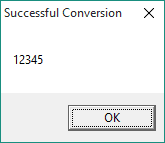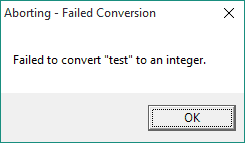Introduction | Example | Tutorial | Applications

## Introduction - CInt

This VBA tutorial shows you how to convert a data type from a string to an integer with the VBA Type Conversion function CInt. Robust error checks included!

This is Part 1 of a new tutorial series showing you how to convert between VBA data types.
Part 2: Convert number to string
Part 3: Convert string to date

## Example - CInt

### Convert a String to an Integer

``````Sub DemoCInt()
Dim i As Integer, str1 As String
str1 = Range("A1")
i = ConvertToInteger(str1)
MsgBox i, , "Successful Conversion"
End Sub

Function ConvertToInteger(v1 As Variant) As Integer
On Error GoTo 100:
ConvertToInteger = CInt(v1)
Exit Function
100:
MsgBox "Failed to convert """ & v1 & """ to an integer.", , "Aborting - Failed Conversion"
End
End Function``````

Make powerful macros with our free VBA Developer Kit

It’s easy to copy and paste a macro like this, but it’s harder make one on your own. To help you make macros like this, we built a free VBA Developer Kit and wrote the Big Book of Excel VBA Macros full of hundreds of pre-built macros to help you master file I/O, arrays, strings and more - grab your free copy below.

I'll take a free VBA Developer Kit

## Tutorial - CInt

The function ConvertToInteger is the heart and soul of this VBA example. You call the function and pass it a string or other numeric value, like I did in the procedure DemoCInt with the command ` i = ConvertToInteger(str1) `.

In the CInt example, str1 was the value in cell “A1,” but you don’t have to pass the function a value stored in a cell. The statement `i = ConvertToInteger("12345")` will work, as well.

If the conversion to an integer succeeds, the value of your string will be changed to an integer and you can use it however you please, including in mathematical expressions.If the conversion fails, a message box will pop up letting you know it failed and your macro execution will abort.### 3 Common Conversion Failures

Why would the conversion fail? There are three common reasons.

1. The first reason the conversion may fail is that your string is not numeric. For example, the string “test” in the image above cannot be converted to an integer. To be numeric, the string must yield a “True” response in the following code `IsNumeric(str1)`.

2. The second common reason for conversion failure is that the numeric value you want to convert is either too large or too small to store as an integer. You see, the integer data type can only store whole numbers between -32,768 and 32,767. Anything outside this range would yield an Overflow error (Run-time error ‘6’). If you want to convert whole numbers outside this range, consider using CLng to convert to a Long data type.

3. The third common reason that CInt conversions fail is that you are attempting to apply the CInt function to an array. Doing this will cause a Type mismatch error.

### Converting Decimals to Integers

Keep in mind that the CInt function rounds decimal numbers. For example, if you are attempting to convert a string or a double data type from “5.5” to an integer, the resulting integer will be reported as a “6.” Likewise, converting a “5.4” will yield an integer of “5.”

## Application Ideas - CInt

The ability to convert VBA data types, especially strings to integers, is handy when your program relies on user input. You will be able to check to see if the user entered a string in the correct format, then you can convert the string to an integer and use it in loops, counters, conditional statements and mathematical expressions.

The CInt Function is just one of several VBA data type conversion functions. I plan to highlight more conversions in future lessons. In the meantime, if you’re looking for more string conversion articles, check out my tutorials on